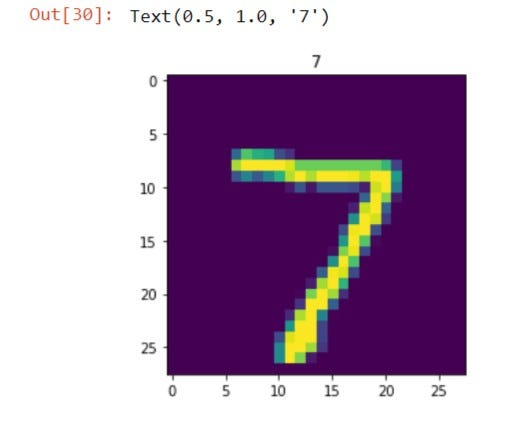# Build neural network in 5 minutes with keras !!!!

Original article was published by Pratik Korat on Artificial Intelligence on Medium# Build neural network in 5 minutes with keras !!!!

Before we get our hand dirty on code make sure that you’ve installed latest version of tensorflow library for python

if you haven’t installed , Don’t Worry i’m posting a link of official documentation that’ll lead you to how to install tensorflow

First of all we’ll import dependencies

`import tensorflow as tffrom tensorflow import kerasimport numpy as npimport matplotlib.pyplot as plt`

we’ll use mnist digit data for classification purpose

`(train_X , train_y),(test_X , test_y)  =   keras.datasets.mnist.load_data()#Reshaping our datatrain_X = train_X.reshape(-1,784)test_X = test_X.reshape(-1,784)#View datashapetrain_X.shape , train_y.shape , train_y.shape , test_y.shape#((60000, 784), (60000,), (60000,), (10000,))`

Now , we will build our Model !!!!

`model = keras.Sequential([    keras.layers.Dense(512 , activation = "relu" , input_dim = 784),    keras.layers.Dropout(0.3),    keras.layers.Dense(10,activation = "softmax")])#We will compile our modelmodel.compile(optimizer = "rmsprop" , loss = keras.losses.SparseCategoricalCrossentropy() , metrics = ["accuracy"])#we will visualize modelmodel.summary()#it will print the summary of your model`

Now , Most interesting part , we’ll start our model’s training….

`r = model.fit(train_X ,train_y,epochs = 10, validation_data = (test_X , test_y) ,batch_size = 256)`

and Boom 💣 🧨 , your model is trained with 0.9696 accuracy..whoa…!!!!

Now , it’s time to check our model

`prediction = model.predict(test_X[:1])plt.imshow(test_X[:1].reshape(28,28))plt.title(np.argmax(prediction))`

That’s all you’ve learned hello world of deep learning…😉😉